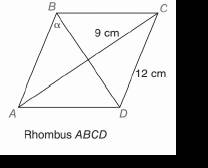Chapter 11.CR, Problem 7CRElementary Geometry For College St...

7th Edition
Alexander + 2 others
ISBN: 9781337614085

Solutions

Chapter
SectionElementary Geometry For College St...

7th Edition
Alexander + 2 others
ISBN: 9781337614085
Textbook Problem

In Review Exercises 5 to 8, state the ratio needed, and use it to find the measure of the indicated angle to the nearest degree.To determine

To find:

The measure of the angle indicated to the nearest degree.

Explanation

Procedure used:

In any right triangle ABC with mA=θ we have the following ratios.

sinθ=OppositeHypotenuse

Calculation:

Given:

The above given figure is a rhombus.

By the properties of a rhombus, all of its sides are equal in length, the diagonals intersect each other at right angle and each of the diagonal bisect the angle at the vertices, we have

AB=BC=CD=DA=12 cm,

mAOB=BOC=COD=DOA=90°, and

mABO=mCBO=α

Here, ‘O’ is the point of intersection of the diagonals AC and BD

Still sussing out bartleby?

Check out a sample textbook solution.

See a sample solution

The Solution to Your Study Problems

Bartleby provides explanations to thousands of textbook problems written by our experts, many with advanced degrees!

Get Started

Evaluate the expression sin Exercises 116. 33

Finite Mathematics and Applied Calculus (MindTap Course List)

Find all degree solutions. tan23=3

Trigonometry (MindTap Course List)

True or False: is a convergent series.

Study Guide for Stewart's Multivariable Calculus, 8th